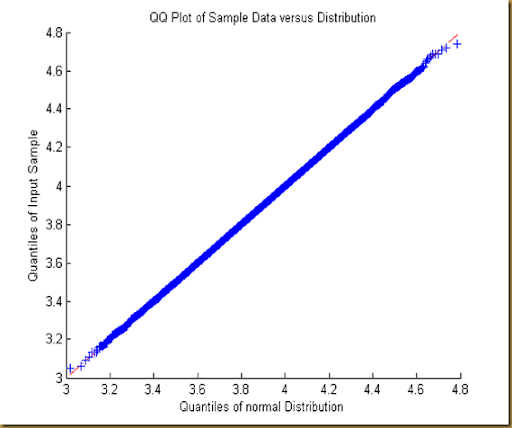## Friday, September 7, 2012

### F#: K-S test on final prices of GBM paths

In the previous post, I mentioned that I chose System.Random, because it’s faster than any other random number generators available in Math.NET Numerics. And I also said that I have a minor concern about System.Random, because someone found a problem in the implementation of System.Random and raised this issue to the .NET team in early 2011. And the team admits it’s a problem. However, this issue is still there with .NET Framework 4.5. And it seems to me that the .NET team is not planning to take any immediate action.

As I know little about the algorithm of System.Random, I’m not sure if it’s worth the efforts to pick up the details and quantify the impact. As such, I decided to take an easy way out, i.e., close my eyes to this issue  do Kolmogrov-Smirnov test (KS test) on only the final prices distribution. If the simulated distribution is statistically indistinguishable from the true, theoretic distribution in terms of shape, I will be fine with System.Random.  At the end of the day, I think what really matters to me is whether System.Random can ultimately give me the correct shape of the final prices distribution I want.

Basically the F# code is pretty much the same as in the previous post except the following:

• I add a run_stat_test function to run the statistical test and generate a Q-Q plot in Matlab.

• I change the Monte Carlo parameter N to 1 so that I simulate only one data point, i.e., final price, for each simulated path.

// Monte Carlo parameters
let T = 1.0             // time horizon in years
let M = 100000          // number of simulations
let N = 1               // number of time intervals

You can see that I take the logarithms of all final prices and test them against a normal distribution with mean = (log S0)+(r-0.5*sigma*sigma)*T and standard deviation = sigma*sqrt(T) using KS test. And the output from the test is as follows:

h = false, p = 0.7243765247

And here is the Q-Q plot:p-value is about 0.72, which means it’s quite likely that the simulated prices were indeed sampled from the normal distribution, and h=false means that we can’t reject the null hypothesis. Moreover, the Q-Q plot does not show any obvious outliers off the diagonal line. Therefore, it’s ok for me to continue to use System.Random.

If any expert happens to know about the issue of System.Random and is willing to shed some light on it, it will be appreciated.

//////////////////////////////////////////////////////////////////////////////////////////////////////////////////////////////////

open MathNet.Numerics.Distributions
open MathNet.Numerics.Statistics

// Monte Carlo parameters
let T = 1.0             // time horizon in years
let M = 100000          // number of simulations
let N = 1               // number of time intervals
let p = 0.99            // confidence level

// underlying dynamics
let S0 = 50.0         // spot price
let sigma = 0.2       // annualized vol
let r = 0.01          // risk-free rate

// risk factor dW
let rnd = new System.Random(1)
let get_dW dt =
let dW = Normal.WithMeanVariance(0.0, dt)
dW.RandomSource <- rnd
(fun () -> dW.Sample())

// GBM path generation
let generate_GBM_paths S0 r sigma T M N =
let dt = T / (float N)
let drift = (r - 0.5 * (sigma**2.0)) * dt
let dW = get_dW dt
Array.init M (fun _ ->
Array.init N (fun _ -> drift + sigma * dW()) |>
Array.scan (+) 0.0 |>
Array.map (fun x -> S0 * exp(x)) )

let run_stat_test (prices:float array) =
let m = (log S0)+(r-0.5*sigma*sigma)*T
let s = sigma*sqrt(T)
let log_prices = Array.map log prices
let matlab = new MLApp.MLAppClass()
matlab.PutWorkspaceData("log_prices", "base", log_prices)
matlab.PutWorkspaceData("m", "base", m)
matlab.PutWorkspaceData("s", "base", s)
matlab.Execute("pd = ProbDistUnivParam('normal',[m s])") |> ignore
matlab.Execute("[h,p] = kstest(log_prices,pd,0.05,0)") |> ignore
matlab.Execute("qqplot(log_prices,pd)") |> ignore
let h = matlab.GetVariable("h","base")
let p = matlab.GetVariable("p","base")
(h,p)

// main
let main () =
let final_prices = generate_GBM_paths S0 r sigma T M N |>
Array.map (fun p -> p.[p.Length - 1])
let forward = final_prices.Mean()
let std_dev = final_prices.StandardDeviation()
let std_error = std_dev / sqrt(float M)
let std_normal = Normal.WithMeanVariance(0.0,1.0)
* std_error
let h,p = run_stat_test final_prices
printfn "h = %A, p = %A" h p
(forward, std_dev, std_error, conf_int)

// run simulation
main()

// calculate theoretical mean and deviation
let true_forward = S0 * exp(r*T)
let v = sigma*sigma*T
let true_std_dev = sqrt((exp(v)-1.0)*exp(2.0*(log(S0)+r*T-0.5*v*T)+v))